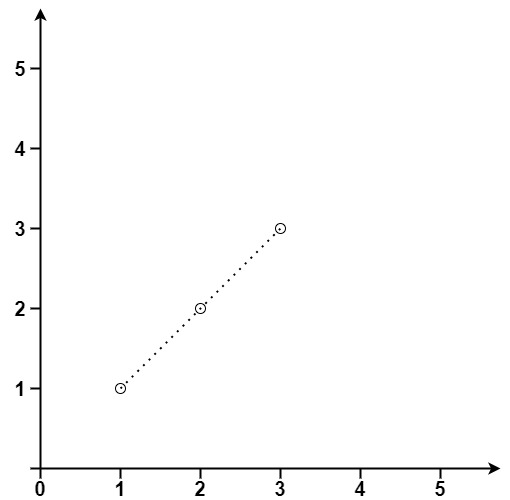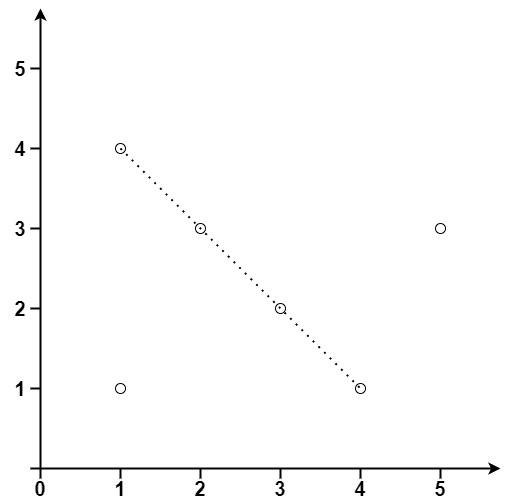# GeetCode Hub

Given an array of `points` where `points[i] = [xi, yi]` represents a point on the X-Y plane, return the maximum number of points that lie on the same straight line.

Example 1:```Input: points = [[1,1],[2,2],[3,3]]
Output: 3
```

Example 2:```Input: points = [[1,1],[3,2],[5,3],[4,1],[2,3],[1,4]]
Output: 4
```

Constraints:

• `1 <= points.length <= 300`
• `points[i].length == 2`
• `-104 <= xi, yi <= 104`
• All the `points` are unique.

class Solution { public int maxPoints(int[][] points) { } }